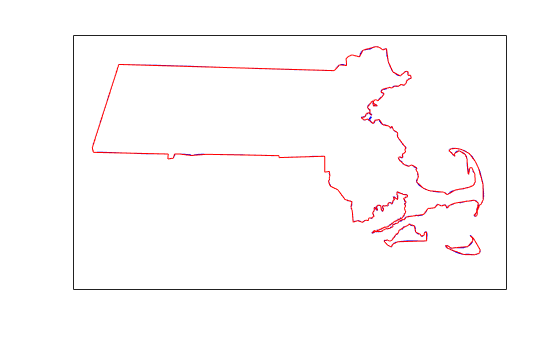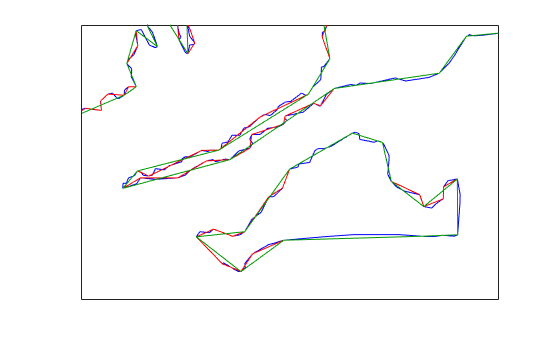Documentation

## Simplify Polygon and Line Data

This example shows how to simplify polygon and line data using the `reducem` function. Simplifying polygon and line data can speed up certain calculations without making any noticeable impact on the data. One way to approach simplification is to use `reducem` with the default tolerance value at first and view the output. If the results do not meet your requirements, repeat the operation, increasing or decreasing the tolerance value to achieve the result you desire. `reducem` returns the tolerance value used. For more information about vector data simplification, see Simplify Vector Coordinate Data.

### Simplify Line Data Using Default Settings

Extract Massachusetts coastlines and state borders from the `usastatehi.shp` shapefile.

```ma = shaperead('usastatehi.shp','UseGeoCoords',true, ... 'Selector', {@(name)strcmpi(name,'Massachusetts'),'Name'}); masslat = ma.Lat; masslon = ma.Lon;```

The result is a pair of vectors that outline the state with 957 vertices.

`numel(masslat)`
```ans = 957 ```

Simplify the outline using the `reducem` function with the default tolerance value.

`[masslat1, masslon1, cerr, tol] = reducem(masslat', masslon');`

Inspect the results. The number of vertices has been reduced to 252. The vectors have been reduced to about a quarter of their original lengths.

`numel(masslat1)`
```ans = 252 ```
`numel(masslat1)/numel(masslat)`
```ans = 0.2633 ```

Examine the error and tolerance values returned by `reducem`. The `cerr` value indicates that `reducem` has reduced the total length of the outline by about 3.3 percent. The tolerance that `reducem` used to achieve this reduction was 0.006 degrees, or about 660 meters.

`[cerr tol]`
```ans = 1×2 0.0331 0.0060 ```

Plot the reduced outline in red over the original outline in blue, using `geoshow`. At this resolution, it's hard to see any difference between the original outline and the reduced outline.

```figure axesm('MapProjection', 'eqdcyl', 'MapLatLim', [41.1 43.0],... 'MapLonLim', [-73.6, -69.8], 'Frame', 'off', 'Grid', 'off'); geoshow(masslat, masslon, 'DisplayType', 'line', 'color', 'blue') geoshow(masslat1, masslon1, 'DisplayType', 'line', 'color', 'red')```To get a better look at the two outlines, use `xlim` and `ylim` to zoom in on a portion of the map. Notice how the reduced outline conforms to the general contours of the original map but loses a lot of the detail.

```axesm('MapProjection', 'eqdcyl', 'MapLatLim', [41.1 43.0],... 'MapLonLim', [-73.6, -69.8], 'Frame', 'off', 'Grid', 'off'); xlim([0.0104 0.0198]) ylim([0.7202 0.7264]) geoshow(masslat, masslon, 'DisplayType', 'line', 'color', 'blue') geoshow(masslat1, masslon1, 'DisplayType', 'line', 'color', 'red')```### Simplify Line Data Changing the Default Tolerance Value

Perform the operation again, this time doubling the tolerance value.

```[masslat2,masslon2,cerr2,tol2] = reducem(masslat', masslon', 0.012); numel(masslat2)```
```ans = 157 ```
`numel(masslat2)/numel(masslat)`
```ans = 0.1641 ```

Examine the error and tolerance values returned by `reducem`. This time, the `cerr` value indicates that `reducem` has reduced the total length of the outline by about 5.2 percent. The tolerance that `reducem` used to achieve this reduction was 0.012 degrees.

`[cerr2 tol2]`
```ans = 1×2 0.0517 0.0120 ```

Plot this reduced outline in dark green over the original outline in blue. Note how this reduced outline maintains the general shape of the original map but loses much of the fine detail.

`geoshow(masslat2, masslon2, 'DisplayType', 'line', 'color', [0 .6 0])`### Simplify Line Data Increasing the Tolerance Again

Increase the tolerance to 0.1 degrees.

`[masslat3, masslon3, cerr3, tol3] = reducem(masslat', masslon', 0.1);`

Plot this reduced outline in black. Note how this reduced map only retains the broadest elements of the original shape and loses much of the detail.

`geoshow(masslat3, masslon3, 'DisplayType', 'line', 'color', 'black')`##### SupportGet trial now